## Jacobi Polynomial

Also known as the Hypergeometric Polynomials, they occur in the study of Rotation Groups and in the solution to the equations of motion of the symmetric top.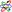They are solutions to the Jacobi Differential Equation. Plugging(1)

into the differential equation gives the Recurrence Relation(2)

for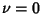, 1, ..., where(3)

Solving the Recurrence Relation gives(4)

for. They form a complete orthogonal system in the intervalwith respect to the weighting function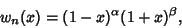(5)

and are normalized according to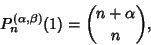(6)

whereis a Binomial Coefficient. Jacobi polynomials can also be written(7)

whereis the Gamma Function and(8)

Jacobi polynomials are Orthogonal satisfying(9)

The Coefficient of the term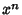in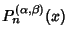is given by(10)

They satisfy the Recurrence Relation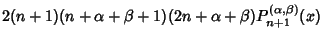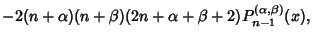(11)
where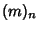is the Rising Factorial(12)

The Derivative is given by(13)

The Orthogonal Polynomials with Weighting Function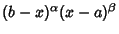on the Closed Interval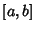can be expressed in the form(14)

(Szegö 1975, p. 58).

Special cases withare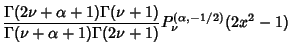(15)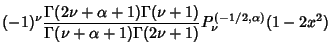(16)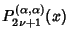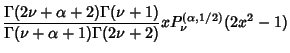(17)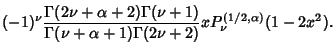(18)

Further identities are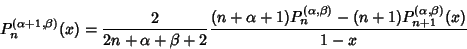(19)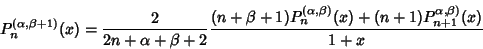(20)(21)
(Szegö 1975, p. 79).

The Kernel Polynomial is(22)
(Szegö 1975, p. 71).

The Discriminant is(23)

(Szegö 1975, p. 143).

For,reduces to a Legendre Polynomial. The Gegenbauer Polynomial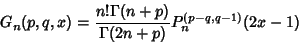(24)

and Chebyshev Polynomial of the First Kind can also be viewed as special cases of the Jacobi Polynomials. In terms of the Hypergeometric Function,(25)(26)

Letbe the number of zeros in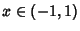,the number of zeros in, and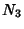the number of zeros in. Define Klein's symbol(27)

where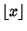is the Floor Function, and(28)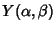(29)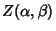(30)

If the cases,, ...,,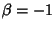,, ...,, and,, ...,are excluded, then the number of zeros ofin the respective intervals are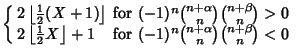(31)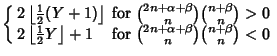(32)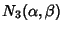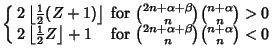(33)

(Szegö 1975, pp. 144-146).

The first few Polynomials are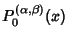(34)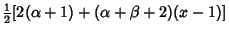(35)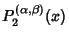(36)

whereis a Rising Factorial. See Abramowitz and Stegun (1972, pp. 782-793) and Szegö (1975, Ch. 4) for additional identities.

See also Chebyshev Polynomial of the First Kind, Gegenbauer Polynomial, Jacobi Function of the Second Kind, Rising Factorial, Zernike Polynomial

References

Abramowitz, M. and Stegun, C. A. (Eds.). Orthogonal Polynomials.'' Ch. 22 in Handbook of Mathematical Functions with Formulas, Graphs, and Mathematical Tables, 9th printing. New York: Dover, pp. 771-802, 1972.

Iyanaga, S. and Kawada, Y. (Eds.). Jacobi Polynomials.'' Appendix A, Table 20.V in Encyclopedic Dictionary of Mathematics. Cambridge, MA: MIT Press, p. 1480, 1980.

Szegö, G. Jacobi Polynomials.'' Ch. 4 in Orthogonal Polynomials, 4th ed. Providence, RI: Amer. Math. Soc., 1975.